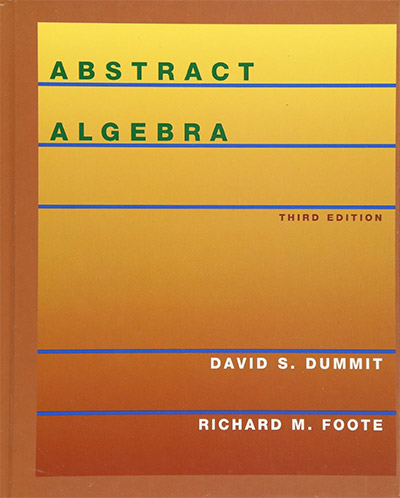# Kernel of Group Homomorphisms

The kernel of a group homomorphism measures how far off it is from being one-to-one (an injection). Suppose you have a group homomorphism f:G → H. The kernel is the set of all elements in G which map to the identity element in H. It is a subgroup in G and it depends on f. Different homomorphisms between G and H can give different kernels. If f is an isomorphism, then the kernel will simply be the identity element. You can also define a kernel for a homomorphism between other objects in abstract algebra: rings, fields, vector spaces, modules. We will cover these in separate videos.

The kernel of a group homomorphism measures how far off it is from being one-to-one (an injection). Suppose you have a group homomorphism f:G → H. The kernel is the set of all elements in G which map to the identity element in H. It is a subgroup in G and it depends on f. Different homomorphisms between G and H can give different kernels. If f is an isomorphism, then the kernel will simply be the identity element. You can also define a kernel for a homomorphism between other objects in abstract algebra: rings, fields, vector spaces, modules. We will cover these in separate videos.

Course Page

## ResourcesAbstract Algebra by Dummit & Foote is a standard textbook used by colleges and universities. It is extremely well written with lots of examples and exercises. It covers all the topics for a solid first course in Abstract Algebra. We use this on occasion as a reference for inspiration or reference. Highly recommended!